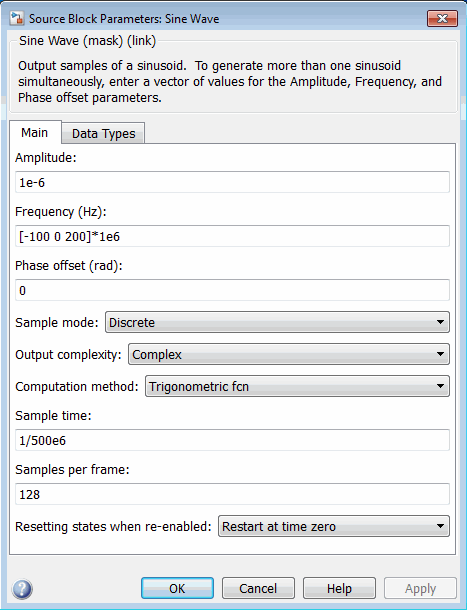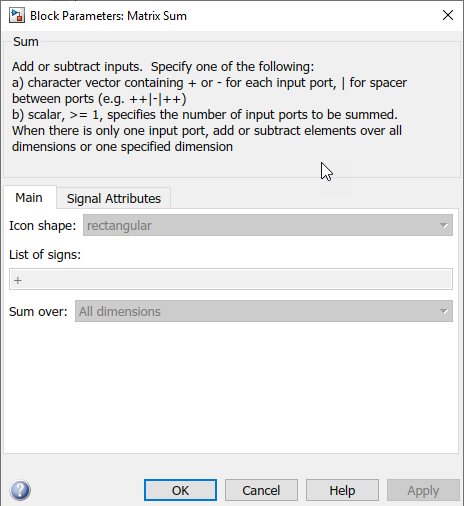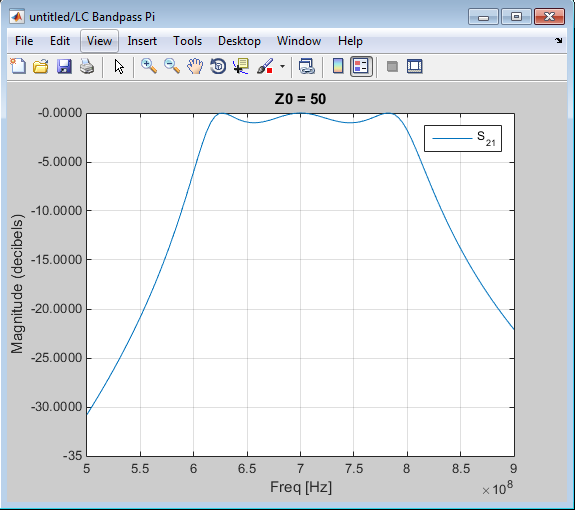## Model RF Filter Using Equivalent Baseband

### Overview of LC Bandpass Filter Example

In this example, you model the signal attenuation caused by an RF filter by comparing the signals at the input and output of the filter.

The RF filter you use in this example is an LC bandpass filter with a bandwidth of 200 MHz, centered at 700 MHz. You use a three-tone input signal to stimulate a range of in-band and out-of-band frequencies of the filter. The input signal has the following tones:

• 700 MHz — Center of the filter

• 600 MHz — Lower edge of the filter passband

• 900 MHz — Outside the filter passband

You simulate the effects of the filter over a bandwidth of 500 MHz.

### Select Blocks to Represent System Components

In this part of the example, you select the blocks to represent the input signal, the RF filter, and the signal displays.

You model the RF filter using a physical subsystem, which is a collection of one or more physical blocks bracketed by an Input Port block and an Output Port block. The RF filter subsystem consists of an LC Bandpass Pi block, and the Input Port and Output Port blocks. The function of the Input Port and Output Port blocks is to bridge the physical part of the model, which uses bidirectional RF signals, and the rest of the model, which uses unidirectional Simulink® signals.

The following table lists the blocks that represent the system components and a description of the role of each block.

Block

Description

Sine Wave

Generates a three-channel signal.

Matrix Sum

Combines the three channel signal into a single three-tone source signal.

Input Port

Establishes parameters that are common to all blocks in the RF filter subsystem, including the source impedance of the subsystem that is used to convert Simulink signals to the RF Blockset™ Equivalent Baseband physical modeling environment.

LC Bandpass Pi

Models the signal attenuation caused by the RF filter which, in this example, is the LC Bandpass Pi filter.

Output Port

Establishes parameters that are common to all blocks in the RF filter subsystem. These parameters include the load impedance of the subsystem, which is used to convert RF signals to Simulink signals.

Spectrum Analyzer

Displays signals at the input to and output of the filter.

### Build the Model

In this part of the example, you create a Simulink model, add blocks to the model, and connect the blocks.

1. Create a model with the blocks shown in the following table. The Library column of the table specifies the hierarchical path to each block.

Block

Library Path

Quantity

Sine WaveDSP System Toolbox > Sources

1

Matrix SumDSP System Toolbox > Math Functions > Matrices and Linear Algebra > Matrix Operations

1

Spectrum AnalyzerDSP System Toolbox > Sinks

2

Input PortRF Blockset > Equivalent Baseband > Equivalent Baseband > Input/Output Ports

1

LC Bandpass PiRF Blockset > Equivalent Baseband > Ladder Filters

1

Output PortRF Blockset > Equivalent Baseband > Input/Output Ports

1

2. Connect the blocks as shown in the following figure.

For more information on connecting physical and mathematical blocks, see Connect Model Blocks.Now you are ready to specify block parameters.

### Specify Model Parameters

In this part of the example, you specify the following parameters to represent the behavior of the system components:

#### Input Signal Parameters

You generate the three-tone source signal using two blocks. You use the Sine Wave block to generate a complex three-channel signal, where each channel corresponds to a different frequency. Then, you use the Matrix Sum block to combine the channels into a single three-tone source signal. Without this block, the signal in all subsequent blocks would have three independent channels.

The RF Blockset Equivalent Baseband simulation algorithm requires you to shift the frequencies of the input signal. The software simulates the filter subsystem using a complex-baseband modeling technique, which automatically shifts the filter response and centers it at zero. You must shift the frequencies of the signals outside the physical subsystem by the same amount.

### Note

All signals in the RF model must be complex to match the signals in the physical subsystem, so you create a complex input signal.

The center frequency of the LC bandpass filter is 700 MHz, so you use a three-tone source signal with tones that are 700 MHz below the actual tones, at -100 MHz, 0 MHz, and 200 MHz, respectively.

1. In Sine Wave block dialog box:

• Set the Amplitude parameter to `1e-6`.

• Set the Frequency (Hz) parameter to `[-100 0 200]*1e6`.

• Set the Output complexity parameter to `Complex`.

• Set the Sample time parameter to `1/500e6`.

• Set the Samples per frame parameter to `128` .2. In the Matrix Sum block dialog box:

• Set the Sum over parameter to ```Specified dimension```.

• Set the Dimension parameter to `2`.#### Filter Subsystem Parameters

In this part of the example, you configure the blocks that model the RF filter subsystem—the Input Port, LC Bandpass Pi, and Output Port blocks.

1. Set the Input Port block parameters as follows:

• Treat input Simulink signal as = ```Incident power wave```

This option tells the blockset to interpret the input signal as the incident power wave to the RF subsystem, and not the source voltage of the RF subsystem.

### Note

If you use the default value for this parameter, the software interprets the input Simulink signal as the source voltage. As a result, the source and the load that model the Input Port and Output Port blocks, respectively, introduce 6 dB of loss into the physical system at all frequencies. For more information on why this loss occurs, see the note in Convert to and from Simulink Signals.

• Center frequency = `700e6`

• Sample time (s) = `1/500e6`

• Input Processing = ```Columns as channels (frame based)```

• Clear the Add noise check box so the software does not include noise in the simulation. To learn how to model noise, see Model Noise.### Note

You must enter the Sample time (s) because the Input Port block does not inherit a sample time from the input signal. The specified sample time must match the sample time of the input signal. The Sample time (s) of `1/500e6` second used in this example is equivalent to a bandwidth of 500 MHz.

2. Accept default parameters for inductance and capacitance in the LC Bandpass Pi block. These parameters create a filter with the desired bandwidth of 200 MHz, centered at 700 MHz.3. Accept the default parameters for the Output Port block to use a load impedance of 50 ohms.#### Signal Display Parameters

In this part of the example, you specify:

1. Set the Spectrum Analyzer parameters as follows:

• In the View tab check Spectrum Settings. In `Trace options ` set the Units parameter to `dBm`.

• In the View tab open Configuration Properties. Set the Minimum Y-limit parameter to `-291` and the Maximum Y-limit parameter to `-67`. Also, set the Y-axis label parameter to `dBm`.

2. Set the Spectrum Analyzer 1 parameters as follows:

• In the View tab check Spectrum Settings. In `Trace options ` set the Units parameter to `dBm`.

• In the View tab open Configuration Properties. Set the Minimum Y-limit parameter to `-291` and the Maximum Y-limit parameter to `-67`. Also, set the Y-axis label parameter to `dBm`.

### Validate Filter Components and Run the Simulation

In this part of the example, you validate the behavior of the LC Bandpass Pi filter block by plotting its frequency response and then run the simulation.

### Note

When you plot information about a physical block, the plot displays the actual frequency response of the block at the selected passband (i.e., the response at the unshifted frequencies), not the response at the shifted frequencies. For more information on this shift, see Input Signal Parameters.

1. Double-click the LC Bandpass Pi block to open the block dialog box.

2. Select the Visualization tab and click Plot to plot the frequency response of the filter. This plots the magnitude of S21 as a function of frequency, which represents the gain of the filter.

Filter Gain### Note

The physical blocks only model a band of frequencies around the center frequency of the physical subsystem. You must choose the sample time and center frequency such that all important frequency characteristics of your physical subsystem fall in this band of frequencies. The plot shows the frequency response of the filter for the portion of the RF spectrum that the physical blocks model. In this example, the physical blocks model a 500-MHz band centered at 700 MHz, as defined by the Input Port block.

3. In the model window, click Run to run the simulation.

### Analyze the Simulation Results

In this part of the example, you analyze the results of the simulation. This section contains the following topics:

#### Compare the Input and Output Signals of the RF Filter

You can view the source signal and the filtered signal in the Spectrum Analyzer windows while the model is running. These windows appear automatically when you start the simulation.

The `Spectrum Analyzer` blocks display the signals at the shifted (baseband-equivalent) frequencies, not at the selected passband frequencies. You can relabel the x-axes of the `Spectrum Analyzer` windows to display the passband signal by entering the Center frequency parameter value of `700e6` (from the Input Port block) for the Frequency display offset (Hz) parameter in the Axis Properties tab of the ```Spectrum Analyzer``` block dialog boxes. For more information on complex-baseband modeling, see Create Complex Baseband-Equivalent Model.

The `Spectrum Analyzer ` blocks display power spectral density normalized to unit sampling frequency. To display power per channel, insert a Gain block with the Gain parameter set to `1/sqrt(N)` before each ```Spectrum Analyzer``` block. `N` is the number of channels. The Gain block is in the Simulink > Commonly Used Blocks library.

In this example, `N` is `128` (the value of the Samples per frame parameter of the Sine Wave block, `128`).

### Note

RF Blockset Equivalent Baseband signals represent amplitudes, not voltages. This means that in the product, power is defined as:

`$Power\text{\hspace{0.17em}}\left(in\text{\hspace{0.17em}}watts\right)\text{\hspace{0.17em}}=\text{\hspace{0.17em}}{\left[Amplitude\text{\hspace{0.17em}}\left(in\text{\hspace{0.17em}}volts/sqrt\left(ohm\right)\right)\right]}^{2}$`

The following plot shows the RF filter input signal you specified in the Sine Wave block.

Input to RF FilterThe next plot shows the filtered signal. Notice the RF filter does not attenuate the signal at the center frequency.

Attenuated Output of RF Filter#### Plot Model Parameters of the Filter Subsystem

After you simulate an RF model, you can evaluate the behavior of the physical subsystem by plotting the network parameters of the Output Port block.

### Note

When you plot information about a physical subsystem, the plot displays the actual frequency response of the subsystem at the selected passband (i.e. the response at the unshifted frequencies), not the response at the shifted frequencies.

To understand the frequency response of the filter, examine the S-parameters as a function of frequency for the RF filter subsystem on a composite plot.

1. Open the dialog box of the Output Port block by double-clicking the block.

2. Select the Visualization tab, and click Plot.

The composite plot, shown in the following figure, contains four separate plots in one figure. For the Output Port block, the composite plot shows the following as a function of frequency (counterclockwise from the upper-left plot):

• An X-Y plane plot of the magnitude of the filter gain, S21, in decibels.

• An X-Y plane plot of the phase of the filter gain, S21, in degrees.

• A Z Smith chart showing the real and imaginary parts of the filter reflection coefficient, S11.

• A Polar plane plot showing the magnitude and phase of the filter reflection coefficient, S11.

### Note

In this example, the response of the filter subsystem is the same as the response of the filter block because the subsystem contains only a filter block.